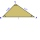# Observer

The observer sees straight fence 100 m long in 30° view angle. From one end of the fence is 153 m.

How far is it from the another end of the fence?

Result

x =  179.9 m

#### Solution:Try calculation via our triangle calculator.

Leave us a comment of example and its solution (i.e. if it is still somewhat unclear...):Be the first to comment!#### To solve this verbal math problem are needed these knowledge from mathematics:

Do you have a linear equation or system of equations and looking for its solution? Or do you have quadratic equation? Do you want to convert length units? See also our trigonometric triangle calculator.

## Next similar examples:

1. RiverFrom the observatory 11 m high and 24 m from the river bank, river width appears in the visual angle φ = 13°. Calculate width of the river.
2. RiverCalculate how many promiles river Dunaj average falls, if on section long 957 km flowing water from 1454 m AMSL to 101 m AMSL.
3. MastMast has 17 m long shadow on a slope rising from the mast foot in the direction of the shadow angle at angle 9.3°. Determine the height of the mast, if the sun above the horizon is at angle 44°30'.
4. Slope of trackCalculate the average slope (in permille and even in degrees) of the rail tracks between Prievidza (309 m AMSL) and Nitrianske Pravno (354 m AMSL), if the track is 11 km long.
5. Gimli GliderAircraft Boeing 767 lose both engines at 42000 feet. The plane captain maintain optimum gliding conditions. Every minute, lose 1910 feet and maintain constant speed 211 knots. Calculate how long takes to plane from engines failure to hit ground. Calculate
6. RectangleThe rectangle is 11 cm long and 45 cm wide. Determine the radius of the circle circumscribing rectangle.
7. Two carsTwo cars started against each other at the same time to journey long 293 km. First car went 41 km/h and second 41 km/h. What distance will be between this cars 20 minutes before meet?
8. ClockHow many times a day hands on a clock overlap?
9. Circle arcCircle segment has a circumference of 135.26 dm and 2096.58 dm2 area. Calculate the radius of the circle and size of central angle.
10. Three altitudesA triangle with altitudes 4; 5 and 6 cm is given. Calculate the lengths of all medians and all sides in a triangle.
11. Cube in a sphereThe cube is inscribed in a sphere with volume 9921 cm3. Determine the length of the edges of a cube.
12. ForcesIn point O acts three orthogonal forces: F1 = 20 N, F2 = 7 N and F3 = 19 N. Determine the resultant of F and the angles between F and forces F1, F2 and F3.
13. TV transmitterThe volume of water in the rectangular swimming pool is 6998.4 hectoliters. The promotional leaflet states that if we wanted all the pool water to flow into a regular quadrangle with a base edge equal to the average depth of the pool, the prism would have.
14. CuboidCuboid with edge a=16 cm and body diagonal u=45 cm has volume V=11840 cm3. Calculate the length of the other edges.
15. Transforming cuboidCuboid with dimensions 6 cm, 10, and 11 cm is converted into a cube with the same volume. What is its edge length?
16. Eiffel TowerEiffel Tower in Paris is 300 meters high, is made of steel. Its weight is 8000 tons. How tall is the tower model made ​​of the same material, if it weigh is 2.4 kg?
17. Triangle ABCCalculate the sides of triangle ABC with area 1404 cm2 and if a: b: c = 12:7:18